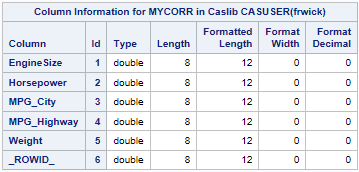A previous article shows how to use the iml action to read a CAS data table into an IML matrix. This article shows how to write a CAS table from data in an IML matrix. You can read an overview of the iml action, which was introduced in SAS Viya 3.5.

### Write a CAS data table from the iml action

Suppose you are running a program in the iml action and you want to write the result of a computation to a CAS data table. The simplest way is to use the the MatrixWriteToCAS subroutine. The syntax of the function is
call MatrixWriteToCAS(matrix, caslib, TableName, colnames);
where

• matrix is the matrix that you want to save.
• caslib is a caslib that specifies the location of the table. A blank string means "use the default caslib." Otherwise, specify the name of a caslib. For example, if the CAS table is in your personal caslib, specify 'CASUSER(userName)', where userName is your login name. For more information, see the CAS documentation about caslibs.
• TableName is a string that specifies the name of the CAS table.
• colnames is a character vector, whose elements specify the columns in the CAS table.

For simplicity, the following call to the iml action defines a matrix and then writes it to a table. In practice, the matrix would be the result of some computation:

```proc cas; loadactionset 'iml'; /* load action set (once per session) */ source WriteMat; corr = {1.0000 0.7874 -0.7095 -0.7173 0.8079, 0.7874 1.0000 -0.6767 -0.6472 0.6308, -0.7095 -0.6767 1.0000 0.9410 -0.7380, -0.7173 -0.6472 0.9410 1.0000 -0.7910, 0.8079 0.6308 -0.7380 -0.7910 1.0000 }; varNames = {'EngineSize' 'Horsepower' 'MPG_City' 'MPG_Highway' 'Weight'}; call MatrixWriteToCas(corr, ' ', 'MyCorr', varNames); /* Write to CAS data table in default caslib */ endsource; iml / code=WriteMat; /* call the iml action to run the program */ run;```

The program writes the matrix to a CAS table named MYCORR. You can call the columnInfo action (which is similar to PROC CONTENTS) to verify that the CAS table exists:

```proc cas; columnInfo / table='MyCorr'; run;```The output of the columnInfo action shows that the MYCORR data table was created. Notice that in addition to the five specified variables, the CAS table contains a sixth variable, called _ROWID_. This variable is automatically added by the MatrixWriteToCAS subroutine.

How did that variable get there? As explained in the previous article, CAS data tables do not have an inherent row order, but matrices do. The iml action includes the _ROWID_ variable in the output data table in case you need to read the data back into an IML matrix at a later time. If you do, the rows of the new matrix will be in the same order as the rows of the CORR matrix that created the data table. For a correlation matrix, this is important because (by convention) the order of the rows corresponds to the order of the columns. Preserving the row order ensures that the matrix has 1s along the main diagonal.

In summary, you can use the MatrixWriteToCAS subroutine to write a matrix to a CAS table. Not only does the call create a CAS table, but it augments the table with information that can recreate the matrix in the same row order. By the way, if you want to save mixed-type data, you can use the TableWriteToCAS subroutine to write a SAS/IML table to a CAS table.